×

Search anything:

# Different built-in data structures in JavaScript

#### JavaScriptGet this book -> Problems on Array: For Interviews and Competitive Programming

JavaScript has a few built-in data structures and in this article at OpenGenus, I will explain their purpose and show examples of their implementations.

1. Arrays
• regular arrays
• typed arrays
2. Sets
• set
• weakset
3. Maps
• map
• weakmap

# 1. Arrays

Arrays are collections of elements. These elements can be strings, numbers, objects, boolean values, or other arrays. Each element has an index (starts with 0) and by referencing their index, we can manipulate the array.
In this article, I will show examples of regular and typed arrays.
First, let's see some common methods with regular arrays.
Regular arrays:

• Creating regular empty arrays
``````const arr1 = [];

const arr2 = new Array(); // []
``````

We can create arrays with the square brackets or with the Array constructor. arr1 and arr2 are both empty arrays at this point.

• Creating regular arrays with elements inside
``````const arr3 = [1, 2, 'string', { color: 'red' }];
``````

Here, I created an array with the square bracket notation and added different types of elements inside of it.

• Create a regular array with empty spaces
``````const arr4 = new Array(5); // [empty, empty, empty, empty, empty]
``````

When we add a number inside the parentheses, it will assign empty spaces inside the array. Now, we can add an element in one of the empty spaces:

``````arr4 = 'string'; // [empty, empty, 'string', empty, empty]
``````

I added the value 'string' at index 2 which is the third place in the array.

• Passing strings and multiple values inside the parentheses
``````const arr5 = new Array('5'); // ['5']

const arr6 = new Array(5, 'cat'); // [5, 'cat']
``````

Here, I added a string instead of a number and this will be added to the array as an element, also, in the second example, passing multiple values in the parentheses will be put inside the array.
Now, let's see some common array methods.

• Insert values at the beginning
``````arr6.unshift('dog'); // ['dog', 5, 'cat']
``````

The unshift() method adds new elements at the front/beginning of the array.

• Insert values at the end
``````arr6.push(4); // ['dog', 5, 'cat', 4]
``````

The push() method adds new elements at the end of the array.

• Insert values at a specific index
``````arr6.splice(1, 0, 'red'); // ['dog', 'red', 5, 'cat', 4]
``````

The splice() method comes in handy when we want to add new elements somewhere between the first and the last elements. Inside the parentheses the number 1 stands for the starting index, so we want to add the new element to the index 1 which is the second place in the array. The number 0 stands for the delete count, but since we don't want to delete anything we will pass the number 0 here, and finally the string 'red' is the value we want to add.

• Delete the first value
``````arr6.shift(); // [ 'red', 5, 'cat', 4]
``````

The shift() method deletes the first element in the array.

• Delete the last value
``````arr6.pop(); // ['red', 5, 'cat']
``````

The pop() method deletes the last element in the array.

• Delete values at a given index
``````arr6.splice(1, 2); // ['red']
``````

Deleting elements between the first and last element in the array is easy with the splice() method. We only need two arguments inside the parentheses, the first one indicates the starting index and the second one indicates the delete count, in this case, we delete 2 elements after the first element.

Typed arrays:
Typed arrays are a little bit different than regular arrays, they are faster but they don't have all the methods that regular arrays have. They are able to read and write raw binary data in memory buffers.
Memory buffer: A memory buffer is like a temporary storage area in the main memory that stores data which is transferring between devices. No matter how fast are these devices are, memory buffers make it possible to complete the tasks and transfer the data between these devices. So for example, if one device is faster than the other one, than it doesn't have to wait for it. Video streaming is a great example where buffers are used.
Why do we need typed arrays?
Typed arrays are really helpful when for example we want to add some video or audio features to our applications and we want to be able to quickly and easily manipulate the raw binary data. Typed arrays separate the implementation into buffers and views. A buffer, which is implemented by the ArrayBuffer object, is an object that represents a chunk of data, however, it doesn't provide access to its contents. To access the data from the buffer, we need to use a view. A view turns the data into an actual typed array.
ArrayBuffer: An ArrayBuffer object is a data type which represents a fixed-length binary data buffer. Since we can't directly manipulate the contents of it, we need to create a typed array view which represents the buffer in a specific format and use that to read and write the contents of the buffer.
Now let's visualize how this works.

1. We create a buffer which is represented by the ArrayBuffer object and it serves as a container for the raw binary data.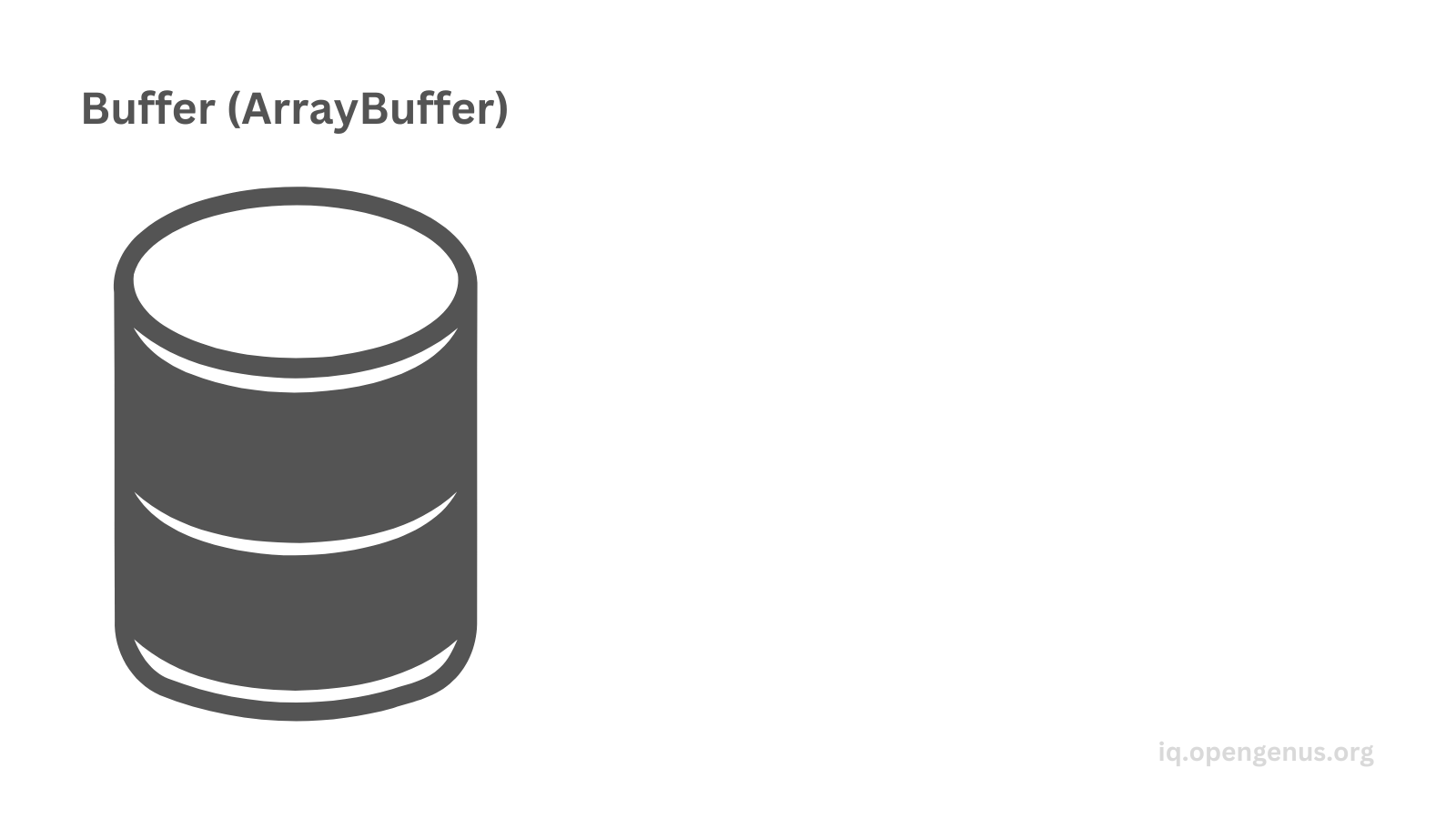2. Then we initialize the buffer with data which can be video or audio content.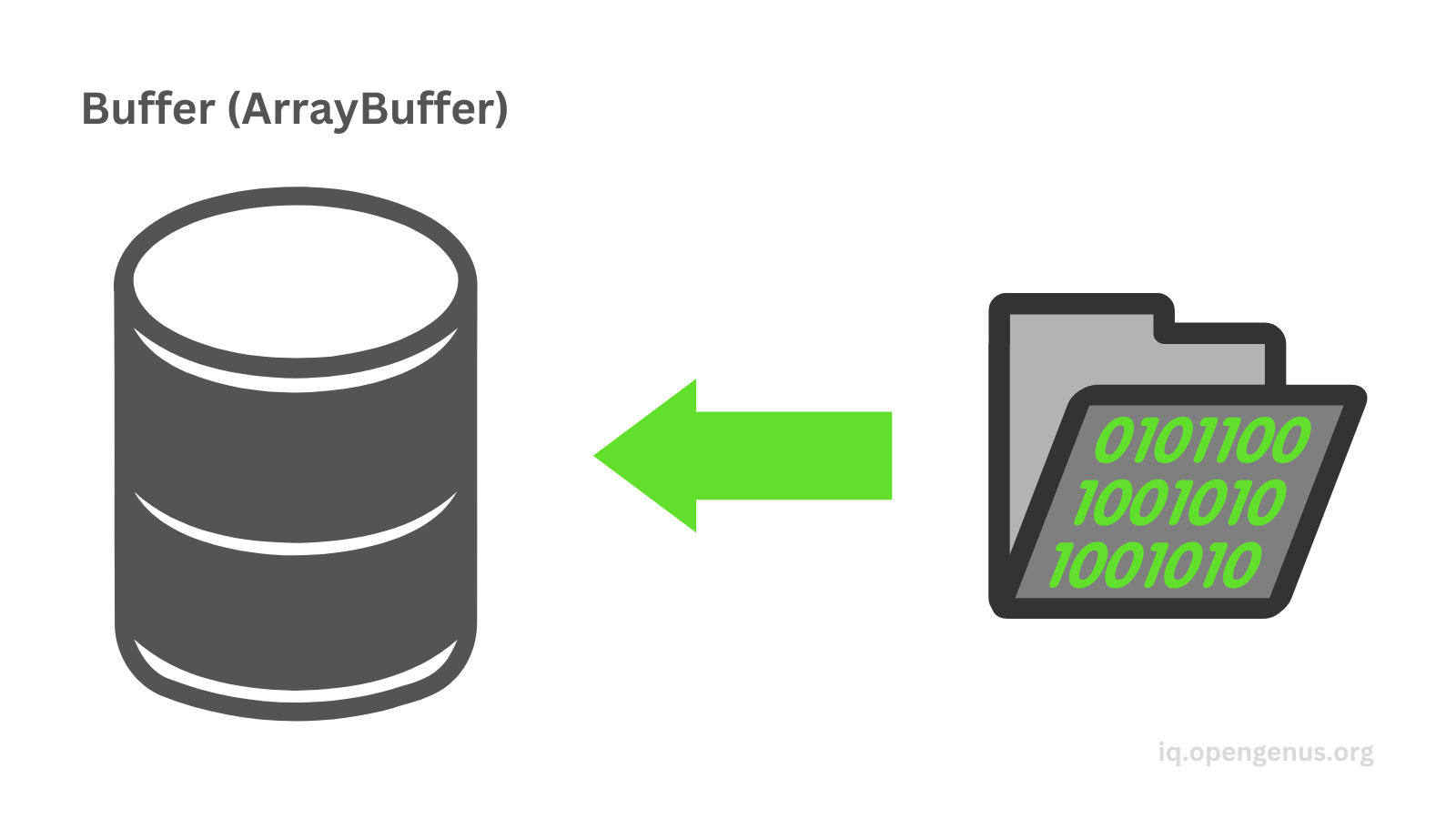3. Then we create a view which is a typed array. A view represents the buffer's contents as a specific type of typed array, for example, UInt8Array, Int16Array, Float32Array, etc.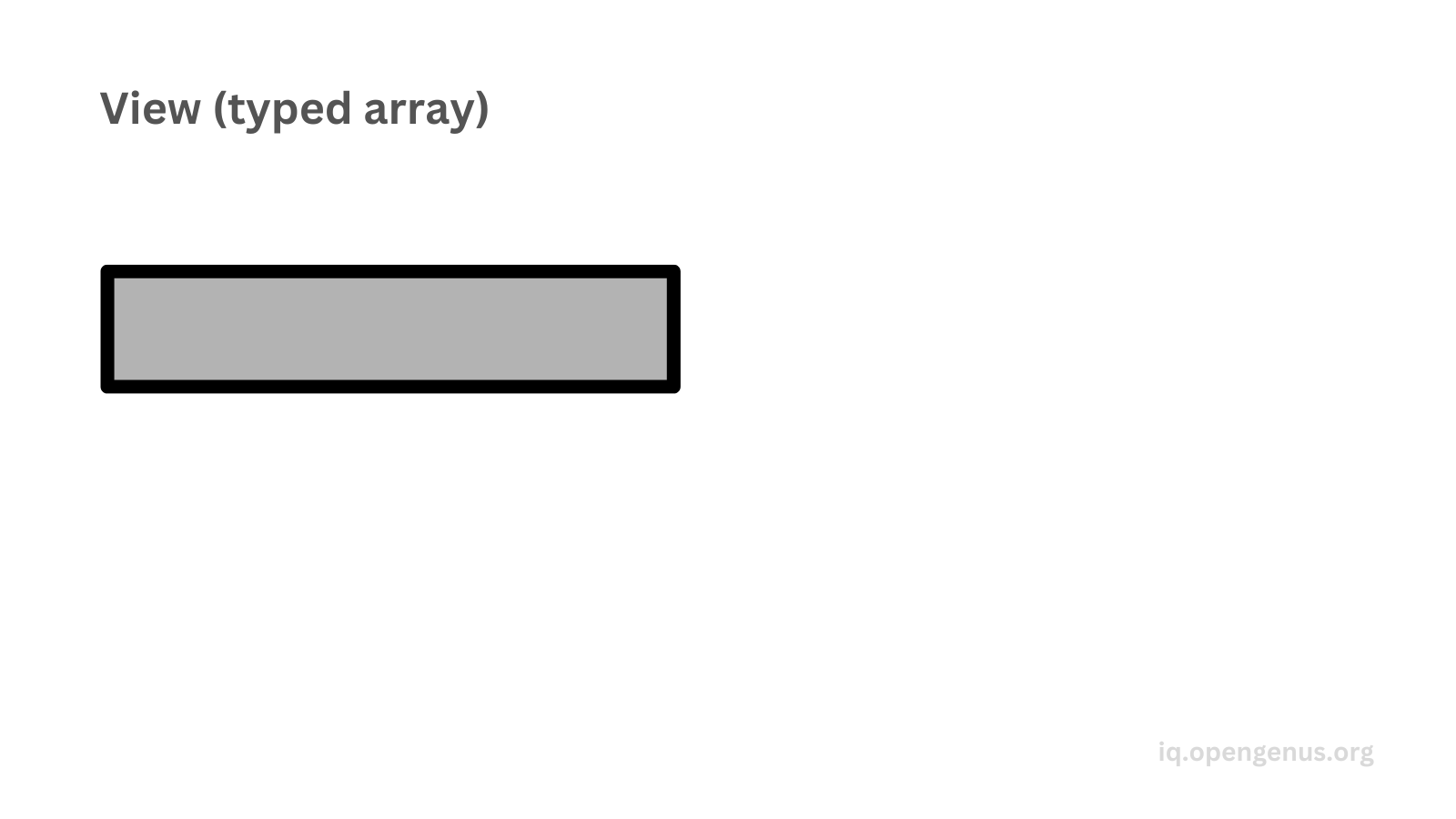4. Then we can manipulate the data. The view provides an interface to access and manipulate the data.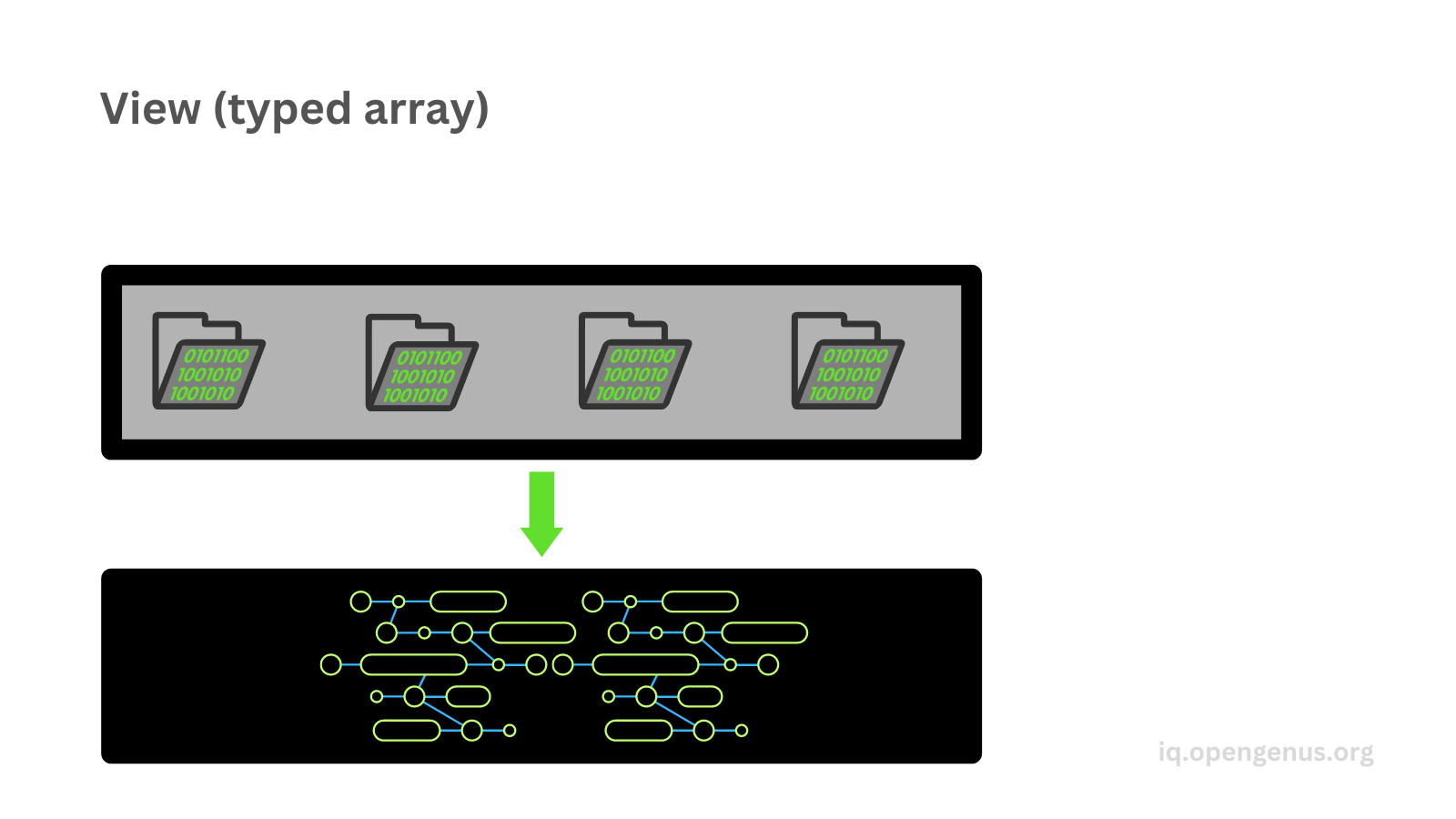5. Finally, we can update the buffer. Any changes we make in the typed array will automatically update the buffer.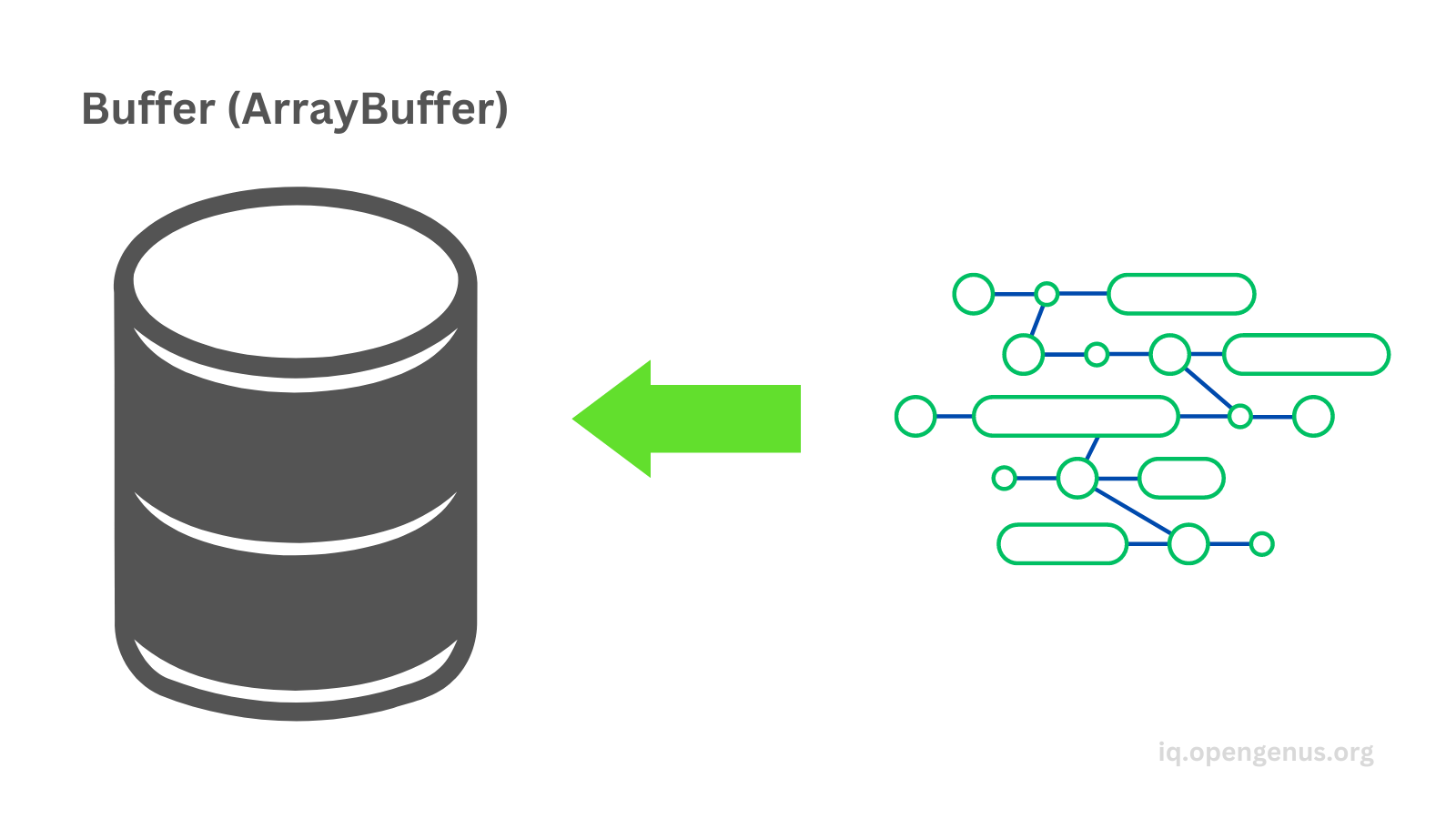Let's see the types of typed arrays:
• Int8Array: Each item is an 8 bit (1 byte) signed integer. These arrays can represent both negative and positive numbers (-128 to 127).
``````const int8Array1 = new Int8Array(); // []
const int8Array2 = new Int8Array(5); // [0, 0, 0, 0, 0]
int8Array2 = 2; // [2, 0, 0, 0, 0]
const bytesPerElement = int8Array2.BYTES_PER_ELEMENT; // 1
``````
• Uint8Array: Each item is an 8 bit (1 byte) unsigned integer. These arrays can only represent positive numbers (0 to 255).
``````const uint8Array1 = new Uint8Array(); // []
const uint8Array2 = new Uint8Array(5); // [0, 0, 0, 0, 0]
uint8Array2 = 5; // [0, 5, 0, 0, 0]
const bytesPerElement2 = uint8Array2.BYTES_PER_ELEMENT; // 1
``````
• Uint8ClampedArray: Represents an array of 8-bit unsigned integers. The difference between Uint8ClampedArray and other typed arrays is that the values are clamped to the range of 0 to 255.
When we try to set a value in a Uint8ClampedArray that is outside the range of 0 to 255, the value is clamped to either 0 or 255, depending on whether it is below or above the valid range. This means that if we try to set a negative value in a Uint8ClampedArray, it will be clamped to 0, and if we try to set a value greater than 255, it will be clamped to 255.
``````const uint8ClampedArray1 = new Uint8ClampedArray(); // []
const uint8ClampedArray2 = new Uint8ClampedArray(3); // [0, 0, 0]
uint8ClampedArray2 = -5; // [0, 0, 0]
uint8ClampedArray2 = 280; // [255, 0, 0]
const bytesPerElement3 = uint8ClampedArray2.BYTES_PER_ELEMENT; // 1
``````
• Int16Array: Represents an array of 16-bit signed integers. This means that each element in the array takes up 2 bytes of memory and can store integer values between -32,768 and 32,767.
``````const int16Array1 = new Int16Array(); // []
const int16Array2 = new Int16Array(3); // [0, 0, 0]
int16Array2 = 20; // [20, 0, 0]
const bytesPerElement4 = int16Array2.BYTES_PER_ELEMENT; // 2
``````
• Uint16Array: Represents an array of 16-bit unsigned integers. This means that each element in the array takes up 2 bytes of memory and can store integer values between 0 and 65,535.
``````const uint16Array1 = new Uint16Array(); // []
const uint16Array2 = new Uint16Array(3); // [0, 0, 0]
uint16Array2 = 24; // [24, 0, 0]
const bytesPerElement5 = uint16Array2.BYTES_PER_ELEMENT; // 2
``````
• Int32Array: Represents an array of 32-bit signed integers. This means that each element in the array takes up 4 bytes of memory and can store integer values between -2,147,483,648 and 2,147,483,647.
``````const int32Array1 = new Int32Array(); // []
const int32Array2 = new Int32Array(3); // [0, 0, 0]
int32Array2 = 50; // [50, 0, 0]
const bytesPerElement6 = int32Array2.BYTES_PER_ELEMENT; // 4
``````
• Uint32Array: Represents an array of 32-bit unsigned integers. This means that each element in the array takes up 4 bytes of memory and can store integer values between 0 and 4,294,967,295.
``````const uint32Array1 = new Uint32Array(); // []
const uint32Array2 = new Uint32Array(3); // [0, 0, 0]
uint32Array2 = 50; // [50, 0, 0]
const bytesPerElement7 = uint32Array2.BYTES_PER_ELEMENT; // 4
``````
• Float32Array: Represents an array of 32-bit floating-point numbers. This means that each element in the array takes up 4 bytes of memory and can store floating-point values with up to 7 significant digits.
``````const float32Array1 = new Float32Array(); // []
const float32Array2 = new Float32Array(3); // [0, 0, 0]
float32Array2 = 2.5; // [2.5, 0, 0]
float32Array2 = -1.5; // [2.5, -1.5, 0]
const bytesPerElement8 = float32Array2.BYTES_PER_ELEMENT; // 4
``````
• Float64Array: Represents an array of 64-bit floating-point numbers. This means that each element in the array takes up 8 bytes of memory and can store floating-point values with up to 15 significant digits.
``````const float64Array1 = new Float64Array(); // []
const float64Array2 = new Float64Array(3); // [0, 0, 0]
float64Array2 = 2.588; // [2.588, 0, 0]
float64Array2 = -1.591; // [2.588, -1.591, 0]
const bytesPerElement9 = float64Array2.BYTES_PER_ELEMENT; // 8
``````
• BigInt64Array: Represents an array of 64-bit signed integers. They are made to handle integers larger than 2^53 - 1.
``````const bigInt64Array1 = new BigInt64Array(); // []
const bigInt64Array2 = new BigInt64Array(3); // [0n, 0n, 0n]
bigInt64Array2 = 2334567n; // [2334567n, 0n, 0n]
const bytesPerElement10 = bigInt64Array2.BYTES_PER_ELEMENT; // 8
``````
• BigUInt64Array: Represents an array of 64-bit unsigned integers. It is made to handle integers larger than 2^53 - 1, but it represents only non-negative integers.
``````const bigUInt64Array1 = new BigUint64Array(); // []
const bigUInt64Array2 = new BigUint64Array(3); // [0n, 0n, 0n]
bigUInt64Array2 = 2334567n; // [2334567n, 0n, 0n]
const bytesPerElement11 = bigUInt64Array2.BYTES_PER_ELEMENT; // 8
``````

# 2. Sets

A set is a collection of data and each data has to be unique (there can be no repeated ones).

Regular sets:

• Creating a new set
``````const set1 = new Set(); // {}
``````
``````set1.add(9); // {9}
const obj1 = { color: 'red', num: 5 };
set1.add(obj1); // {9, 'string', {color: 'red', num: 5}}
``````
• Checking if it has a certain item with the has() method
``````const hasTheNumber9 = set1.has(9); // true
const hasTheStringDog = set1.has('dog'); // false
``````
• Getting the size of the set with the size property
``````const sizeOfSet = set1.size; // 3
``````
• Deleting an item with the delete() method
``````set1.delete(obj1); // {9, 'string'}
``````

We can't delete objects from it directly, the following will not work:

``````const set2 = new Set();
set2.add({ color: 'blue' }); // {3, {color: 'blue'}}
set2.delete({ color: 'blue' });
console.log(set2); // {3, {color: 'blue'}}
``````

When we remove an object outside of the set, then the object still remains in the set:

``````let obj2 = { color: 'green' };
set2.add(obj2); // {3, {color: 'blue'}, {color: 'green'}}
obj2 = null;
console.log(set2); // {3, {color: 'blue'}, {color: 'green'}}
``````

Weaksets:
They are a collection of garbage-collectable objects. If an object that is stored in a weakset is no longer referenced by any other part of the code, it will be automatically removed from the weakset. They don't have the size property and they are not iterable.

``````const weakSet = new WeakSet(); // {}
const obj3 = { fruit: 'apple' };
const obj4 = { fruit: 'apple' };
let hasObj3 = weakSet.has(obj3); // true
let hasObj4 = weakSet.has(obj4); // true
weakSet.delete(obj3);
hasObj3 = weakSet.has(obj3); // false
``````

Another approach of declaring a weakset:

``````let obj5 = { animal: 'bear' };
let obj6 = { color: 'yellow' };
let obj7 = { pet: 'cat' };
const weakSet2 = new WeakSet([obj5, obj6, obj7]); // {}
``````

When an object is removed outside of a weakset, then it will be automatically removed by the weakset:

``````let hasObj5 = weakSet2.has(obj5); // true
obj5 = null;
hasObj5 = weakSet2.has(obj5); // false
``````

# 3. Maps

Maps hold key-value pairs where a key may occur only once. Any data type can be either a key or a value.

Regular maps:

• Creating maps and adding items with the set() method
``````const map1 = new Map(); // {}
map1.set('color', 'red');
map1.set({ obj: 'an object' }, true);
map1.set(1, 'number');

console.log(map1); //  {'color' => 'red', { obj: 'an object' } => true, 1 => 'number'}
``````
• Deleting items with the delete() method
``````map1.delete('color');
console.log(map1); // {{ obj: 'an object' } => true, 1 => 'number'}
``````
• Checking if it contains a specific item with the has() method
``````const hasKey1 = map1.has(1); // true
``````
• Getting the value of a specific key with the get() method
``````const getValueOfKey1 = map1.get(1); // 'number'
``````

Weakmaps:
They are a collection of key-value pairs whose keys must be objects only. All the characteristics from the weakset are true for weakmaps, they will automatically remove the object if it's not referenced by any other part of the code, they don't have a size property and they are not iterable eaither.

``````const weakMap = new WeakMap(); // {}
const obj1 = { color: 'black' };
const obj2 = {};
weakMap.set(obj1, 'dark'); // {}
weakMap.set(obj2, 12); // {}

const hasTheValue12 = weakMap.has(obj2); // true
const getTheValue = weakMap.get(obj1); // 'dark'

weakMap.delete(obj1);
const hasTheValueDark = weakMap.has(obj1); // false
``````